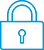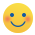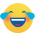cancel
Showing results for
Did you mean:It has been a while since anyone has replied. Simply ask a new question if you would like to start the discussion again.New member
1 0 0 0
Message 1 of 6
918
Flag Post

# Graphing an astroid curveHP Recommended
50G Graphing calculator

the function I would like  to graph is  x^(2/3) +y^(2/3) = 4

Is my difficult the fact that the exponent is not n integer?

Thank you

Tags (3)
5 REPLIES 5Level 12
11,043 10,554 577 1,703
Message 2 of 6
Flag PostHP Recommended

>  I would like  to graph is  x^(2/3) +y^(2/3) = 4

What happens if you graph:   2/3*log(x) + 2/3*log(y) = log(4) ?

Or:  log(x) + log(y) = 1.5* log(4) ?

By the way, is this a "homework" assignment?

If so, please give credit to this forum for helping you with your school-work.Level 6
305 297 32 76
Message 3 of 6
Flag PostHP Recommended

@john229 wrote:

the function I would like  to graph is  x^(2/3) +y^(2/3) = 4

Is my difficult the fact that the exponent is not n integer?

Thank you

Hello,

What did you choose forType in 2D/3D settings? Function or Conic?

Your equation is not in the form of a function, because a function is in the form:  y=f(x)

In my opinion for Conic plotting the exponent of y has to be 1 or 2, so it is not usable either.

But you can rewrite your equation in the form of a function:

y=[4-x^(2/3)]^3/2

and this you can plot.Level 12
11,043 10,554 577 1,703
Message 4 of 6
Flag PostHP Recommended

>   log(x) + log(y) = 1.5* log(4)

Thus,   log(y) = 1.5* log(4) - log(x)

Thus, y = exp((1.5*log(4)-log(x)))

to cast it as y = function(x)Level 3
26 23 1 5
Message 5 of 6
Flag PostHP Recommended

The log of a sum is not equal to the sum of the logs. The log of a product is the sum of the logs.Level 12
11,043 10,554 577 1,703
Message 6 of 6
Flag PostHP Recommended

Indeed.If it was a "homework" question, then copying an incorrect answer from the Internet will be marked as incorrect.

Moral: learn the material -- don't believe everything you read on the Internet.† The opinions expressed above are the personal opinions of the authors, not of HP. By using this site, you accept the Terms of Use and Rules of Participation